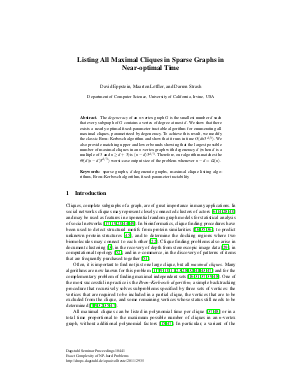Document# Listing all maximal cliques in sparse graphs in near-optimal time

### Authors David Eppstein, Maarten Löffler, Darren Strash## File

DagSemProc.10441.2.pdf
• Filesize: 405 kB
• 14 pages

## Cite As

David Eppstein, Maarten Löffler, and Darren Strash. Listing all maximal cliques in sparse graphs in near-optimal time. In Exact Complexity of NP-hard Problems. Dagstuhl Seminar Proceedings, Volume 10441, pp. 1-14, Schloss Dagstuhl - Leibniz-Zentrum für Informatik (2011)
https://doi.org/10.4230/DagSemProc.10441.2

## Abstract

The degeneracy of an \$n\$-vertex graph \$G\$ is the smallest number \$d\$ such that every subgraph of \$G\$ contains a vertex of degree at most \$d\$. We show that there exists a nearly-optimal fixed-parameter tractable algorithm for enumerating all maximal cliques, parametrized by degeneracy. To achieve this result, we modify the classic Bron--Kerbosch algorithm and show that it runs in time \$O(dn3^{d/3})\$. We also provide matching upper and lower bounds showing that the largest possible number of maximal cliques in an \$n\$-vertex graph with degeneracy \$d\$ (when \$d\$ is a multiple of 3 and \$nge d+3\$) is \$(n-d)3^{d/3}\$. Therefore, our algorithm matches the \$Theta(d(n-d)3^{d/3})\$ worst-case output size of the problem whenever \$n-d=Omega(n)\$.
##### Keywords
• Clique
• backtracking
• degeneracy
• worst-case optimality

## Metrics

• Access Statistics
• Total Accesses (updated on a weekly basis)
0